## Vector Spherical Harmonic

The Spherical Harmonics can be generalized to vector spherical harmonics by looking for a Scalar Function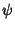and a constant Vectorsuch that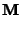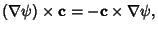(1)

so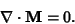(2)

Now use the vector identities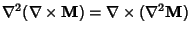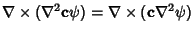(3)(4)

so(5)

andsatisfies the vector Helmholtz Differential Equation ifsatisfies the scalar Helmholtz Differential Equation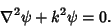(6)

Construct another vector function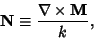(7)

which also satisfies the vector Helmholtz Differential Equation since(8)

which gives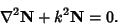(9)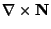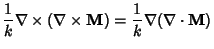(10)

In this formalism,is called the generating function andis called the Pilot Vector. The choice of generating function is determined by the symmetry of the scalar equation, i.e., it is chosen to solve the desired scalar differential equation. Ifis taken as(11)

where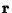is the radius vector, thenis a solution to the vector wave equation in spherical coordinates. If we want vector solutions which are tangential to the radius vector,(12)

so(13)

and we may take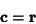(14)

(Arfken 1985, pp. 707-711; Bohren and Huffman 1983, p. 88).

A number of conventions are in use. Hill (1954) defines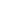(15)(16)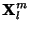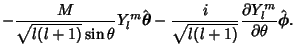(17)

Morse and Feshbach (1953) define vector harmonics called,, and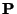using rather complicated expressions.

References

Arfken, G. Vector Spherical Harmonics.'' §12.11 in Mathematical Methods for Physicists, 3rd ed. Orlando, FL: Academic Press, pp. 707-711, 1985.

Blatt, J. M. and Weisskopf, V. Vector Spherical Harmonics.'' Appendix B, §1 in Theoretical Nuclear Physics. New York: Wiley, pp. 796-799, 1952.

Bohren, C. F. and Huffman, D. R. Absorption and Scattering of Light by Small Particles. New York: Wiley, 1983.

Hill, E. H. The Theory of Vector Spherical Harmonics.'' Amer. J. Phys. 22, 211-214, 1954.

Jackson, J. D. Classical Electrodynamics, 2nd ed. New York: Wiley, pp. 744-755, 1975.

Morse, P. M. and Feshbach, H. Methods of Theoretical Physics, Part II. New York: McGraw-Hill, pp. 1898-1901, 1953.